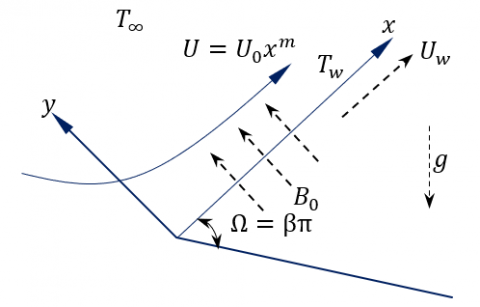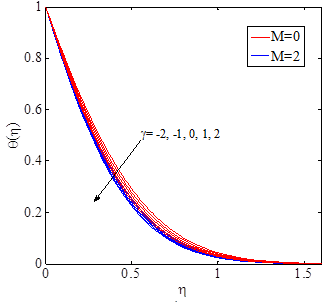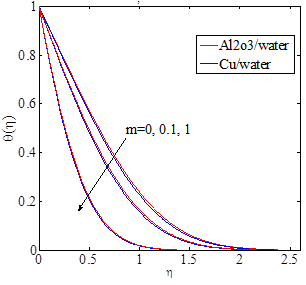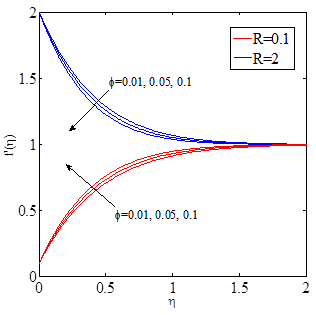# MHD Mixed Convective Heat Transfer of Cu-Al2O3 Water Hybrid Nanofluid over a Stretching Wedge with Ohmic Heating

MHD Mixed Convective Heat Transfer of Cu-Al2O3 Water Hybrid Nanofluid over a Stretching Wedge with Ohmic Heating

Department of Mathematics, Faculty of Science, South Valley University, Qena 82325, Egypt

Department of Mathematics, Faculty of Science, Taibah University, P.O. Box 344 Al-Madinah Al-Munawara, Saudi Arabia

Department of Mathematics, Faculty of Science, Aswan University, Aswan 81528, Egypt

Corresponding Author Email:
tarabi@taibahu.edu.sa
Page:
475-481
|
DOI:
https://doi.org/10.18280/ijht.400215
27 December 2021
|
Accepted:
16 March 2022
|
Published:
30 April 2022
| Citation

OPEN ACCESS

Abstract:

The main purpose of the current article is present exploration scrutinizes a numerical simulation for the magneto-mixed convection flows of hybrid nanofluid over a stretching wedge with Ohmic heating applying Tiwari and Das model. Proper dimensionless variables are applied the governing PDEs which are solved numerically using Matlab program. The obtained results of velocity and temperature profiles for several key parameters are portrayed and construed in detail. It has been observed that the amount of skin friction coefficient and the Nusselt number of the hybrid liquid nano improve and increase with the increase of the nanoparticle size fraction, magnetic field, mixed load, and wedge angle while it decreases when we increase the velocity ratio and increases with the increase of the Nusselt number.

Keywords:

mixed convection, MHD, hybrid nanofluid, ohmic heating

1. Introduction

Mixed convection flow occurs when natural convection and forced convection technique work with each other to transfer heat. The study of the flow and heat transfer has great practical applications in the field of technology and science. Mixed convection flow over a stretching surface is one of these studies that play an important role in engineering, petroleum and agriculture industries like ion exchange columns, heat-treated materials operating between rolls or on a conveyor belt, materials production by extrusion processes, distillation towers, solar power absorbers and subterranean chemical waste migration etc. [1-9] are some of the studies that concentrate on the mixed convective flow over a perpendicular stretching surface in various cases. The study of the magnetic properties and behavior of electrically conducting fluids is called "Magnetohydrodynamics". This word has come from magneto which means magnetic field, hydro- which means water, and dynamics which mean movement. Saltwater, plasmas, liquid metals and electrolytes are examples of such magnetofluids. Alfvén  who is starting MHD. Mahdy and Nabwey  reported a numerical analysis of buoyancy-driven nanoliquid flow of gyrotactic time-mixed bioconvection heat transfer in stagnation domain of an impulsively revolving sphere due to convective boundary condition. Ahmed and Mahdy  delignated the case of mixed convection flow through an isothermal vertical wedge submersed in saturated porous medium using Buongiorno's nanofluid.

Magnetic ﬁelds are vastly utilized in modern metallurgical and metal-working processes like MHD power generators, the cooling of reactors, ﬂow meters and MHD pumps etc. Magnetohydrodynamic debates the induced magnetic features of the electrical conductive fluids. In general, its impact on the stream and heat transfer knows as Lorentz force and Joule heating. Actually, exerting the magnetic field provides the resistance in the stream course and reduce the fluid motion. MHD mixed convective ﬂow over a perpendicular stretching surface has been checked by Kumari and Nath  and Ishak et al. . MHD mixed convection ﬂow in an upper sided lid-driven cavity heated by a corner heater which was considered by Oztop et al. . The number of studies associated with mixed convection flow over a wedge is very limited. The numerical results for mixed convection ﬂow over a wedge with variable surface temperature were given by Hossain et al. . The MHD mixed convective mass and heat transfer over a permeable wedge in the existence of a chemical reaction was checked by Muhaimin et al. . MHD mixed convective mass and heat transfer past a permeable wedge was studied by Kandasamy et al.  and took into the effects of chemical reaction, thermophoresis, and variable viscosity.

2. Mathematical Formulation

Table 1. Thermo-physical properties of Copper and Alumina [29, 30]

 Property Water Copper Cu Alumina Al2O3 $\rho$ Cp k $\beta$ $\sigma$ $\mu$ 997.1 4179 0.613 21x10-5 0.05 8.9x10-4 8933 385 401 1.67x10-5 5.96x107 -- 3970 765 40 0.85x10-5 1x10-10 --

## 1.pngFigure 1. Flow model

Consider the problem of steady 2D magneto-hydrodynamic mixed convection flow of hybrid nanofluid Cu-Al2O3 water and heat transfer over a stretching wedge in an animated viscous, incompressible and electrically conducting ﬂuid. As shown from Figure 1, the x-axis is been selected to be the along the wedge surface, and the normal to it is the y-axis. $U=U_{0} x^{m}$ is the velocity of the stream flow far away from the wedge. Uw=UR is the velocity of the stretching of the wedge, were $(R \geq 0). B(x)=B_{0} x^{(m-1) / 2}$ is the magnetic field and applied in the y-direction. The induced magnetic ﬁeld is neglected due to the assuming that the magnetic Reynolds number is small. The temperature in the external side of the temperature boundary layer is $T_{\infty}$. The stretching wedge surface temperature is $T_{w}=T_{\infty}+b x^{2 m}. \beta=2 m /(m+1)$, is the angle parameter of the wedge and $\Omega=\beta \pi$ for an overall angle of the wedge. $\beta=0$ in the case of the wall is horizontal and $\beta=1$ in the case of the wall is perpendicular. Table 1 shows the properties of thermophysical of the nanoparticles and base fluid [29, 30]. By these assumptions, the equations governing can be written as:

Continuity equation

$\frac{\partial u}{\partial x}+\frac{\partial v}{\partial y}=0$                     (1)

Momentum equation

$u \frac{\partial u}{\partial x}+v \frac{\partial u}{\partial y}=U \frac{d U}{d x}+v_{h n f}\left(\frac{\partial^{2} u}{\partial y^{2}}\right)+g \beta_{h n f}\left(T-T_{\infty}\right) \sin \frac{\Omega}{2}-\frac{\sigma_{h n f} \,\,B^{2}}{\rho_{h n f}}(u-U)$                     (2)

Energy equation

$\left(\rho C_{p}\right)_{h n f}\left(u \frac{\partial T}{\partial x}+v \frac{\partial T}{\partial y}\right)=-\rho_{h n f} u\left(U \frac{d U}{d x}+\frac{\sigma_{h n f}}{\rho_{h n f}} B_{0}{ }^{2} U\right)+\alpha_{h n f}\left(\frac{\partial^{2} T}{\partial y^{2}}\right)+\sigma_{h n f} B^{2} u^{2}$                       (3)

with the boundary conditions

$\left.\begin{array}{c}y=0: u(x, y)=U_{w}, v(x, y)=0 \\ T=T_{w}(x)=T_{\infty}+b x^{2 m} \\ y \rightarrow \infty: u(x, y)=U(x), T(x, y)=T_{\infty},\end{array}\right\}$             (4)

where, u is the component of the velocity along x-direction and v is the component of the velocity along the y-direction. T is the temperature, g is the gravity ﬁeld, $\beta_{h n f}$ is the thermal expansion coefficient, $\left(\rho c_{p}\right)_{h n f}$ is the speciﬁc heat at a fixed pressure, $\mu_{h n f}$ is the viscosity of the hybrid nanoﬂuid, $\alpha_{h n f}$ is the thermal conductivity of the hybrid nanoﬂuid, $v_{h n f}=\mu_{h n f} / \rho{ }_{h n f}$ is the kinematics viscosity of the hybrid nanoﬂuid.

We can define some of the similarity transformations as:

$\left.\begin{array}{c}\psi(x, \eta)=\sqrt{U x v_{f}} f(\eta) \\ \eta=y \sqrt{\frac{U}{v_{f} x}}, \theta(x, \eta)=\frac{T-T_{\infty}}{T_{w}-T_{\infty}}\end{array}\right\}$                (5)

where, $\theta$ is the dimensionless temperature, $f^{\prime}=u / U$ is the dimensionless velocity.

By substituting from Eq. (5) into Eq. (1:3) we get:

$f^{\prime \prime \prime}=\frac{\sigma_{h n f}}{\sigma_{f}}(1-\varphi)^{2.5} \mathrm{M}\left(f^{\prime}-1\right)-\frac{\rho_{h n f}}{\rho_{f}}(1-\varphi)^{2.5}\left(\frac{m+1}{2} f f^{\prime \prime}+m\left(1-f^{\prime 2}\right)+\frac{\beta_{h n f}}{\beta_{f}} \gamma\left(\sin \frac{\Omega}{2}\right) \theta\right)$                         (6)

$\theta^{\prime \prime}=\operatorname{Pr}\left(\frac{k_{h n f}}{k_{f}} \frac{\left(\rho C_{p}\right)_{f}}{\left(\rho C_{p}\right)_{h n f}}\right)^{-1}\left(\frac{\left(\rho C_{p}\right)_{h n f}}{\left(\rho C_{p}\right)_{f}}\left(-\frac{m+1}{2} f \theta^{\prime}+2 m f^{\prime} \theta\right)-E c\left(M \frac{\sigma_{h n f}}{\sigma_{f}} f^{\prime 2}-\left(m \frac{\rho_{h n f}}{\rho_{f}}+\frac{\sigma_{h n f}}{\sigma_{f}} M\right) f^{\prime}\right)\right)$                       (7)

The factors appeared are $\gamma=\frac{G r_{x}}{R e^{2} x}$, $\mathrm{M}=\frac{\sigma_{f} B_{0}^{2}}{\rho_{f} U_{0}}$, $\operatorname{Pr}=\frac{\mu_{f}\left(C_{p}\right)_{f}}{k_{f}}$, $R e_{x}=\frac{U x}{v_{f}}$, $E c=\frac{U^{2}}{\left(C_{p}\right)_{f}\left(T_{w}-T_{\infty}\right)}$, $G r_{x}=\frac{g \beta_{0} f\left(T_{w}-T_{\infty}\right) x^{3}}{v_{f}^{2}}$.

where, $\gamma$ is the mixed convection parameter that refers to the effect of the thermal buoyancy force on the field of the flow, Pr is the Prandtl number, Ec is Eckert number, M is the magnetic parameter, Grx is local Grashof number, Rex is local Reynolds number.

The boundary conditions which defined in Eq. (4), after transformations become:

$\left.\begin{array}{c}f(0)=0, f^{\prime}(0)=R, f^{\prime}(\infty)=1 \\ \theta(0)=1, \theta(\infty)=0,\end{array}\right\}$                     (8)

where, R is the velocity ratio, when R>1 the wedge stretching is faster, when R<1 the wedge stretching slower. The prime refers to the derivative with respect to $\eta$. The physical quantities of interest, local Nusselt number and local skin friction coeﬃcient are defined as:

Skin friction coefficient and Nusselt number are

$C_{f}=\frac{\mu_{h n f}\left(\frac{\partial u}{\partial y}\right)_{y=0}}{\rho_{f} U_{w}^{2}}$

$N u_{x}=-\frac{x k_{h n f}\left(\frac{\partial T}{\partial y}\right)_{y=0}}{k_{f}\left(T_{w}-T_{\infty}\right)}$

Hence

$R e_{x}^{1 / 2} C_{f}=\frac{f^{\prime \prime}(0)}{(1-\varphi)^{2.5}}$

$R e_{x}^{-1 / 2} N u_{x}=-\frac{k_{h n f}}{k_{f}} \theta^{\prime}(0)$                         (9)

3. Thermo-Physical Properties of Regular Nanofluids and Hybrid Nanofluids

The equation of density in nanofluid is:

$\rho_{n f}=(1-\varphi) \rho_{f}+\varphi \rho s \rho_{n f}=(1-\varphi) \rho_{f}+\varphi \rho_{s}$

And in Hybrid nanofluid is:

$\rho_{h n f}=\left(1-\left(\varphi_{s 1}+\varphi_{s 2}\right)\right) \rho_{f}+\varphi_{s 1} \rho_{s 1}+\varphi_{s 2} \rho_{s 2}$

The effective dynamic viscosity of the nanofluid according to the Brinkman model  is given by

$\mu_{n f}=\frac{\mu_{f}}{(1-\varphi)^{2.5}}$

where, $\mu_{f}$ is the viscosity of the fluid fraction, so the effective dynamic viscosity of hybrid nanofluid is

$\mu_{h n f}=\frac{\mu_{b f}}{\left(1-\left(\varphi_{s 1}+\varphi_{s 2}\right)\right)^{2.5}}$

The equation of heat capacity in nanofluid is

$\left(\rho c_{p}\right)_{n f}=(1-\varphi)\left(\rho c_{p}\right)_{f}+\varphi\left(\rho c_{p}\right)_{s}$

The equation of heat capacity in hybrid nanofluid is:

$\left(\rho_{C p}\right)_{h n f}=(1-\varphi)\left(\rho c_{p}\right)_{f}+\varphi_{s 1}\left(\rho c_{p}\right)_{s 1}+\varphi_{s 2}\left(\rho c_{p}\right)_{s 2}$

The equation of thermal conductivity in nanofluid for spherical nanoparticles and according to the Maxwell-Garnetts model  is defined as:

$\frac{k_{n f}}{k_{f}}=\frac{\left(k_{s}+2 k_{f}\right)-2 \varphi\left(k_{f}-k_{s}\right)}{\left(k_{s}+2 k_{f}\right)+\varphi\left(k_{f}-k_{s}\right)}$

From this, the thermal diffusivity of nanofluid defined as:

$\alpha_{n f}=\frac{k_{n f}}{\left(\rho C_{p}\right)_{n f}}$

The equation of thermal conductivity in hybrid nanofluid if thermal conductivity is defined as according to the Maxwell-Garnetts model should give by

$\frac{k_{h n f}}{k_{f}}=\frac{\frac{\varphi_{s 1} k_{s 1}+\varphi_{s 2} k_{s 2}}{\varphi}+2 k_{f}+2\left(\varphi_{s 1} k_{s 1}+\varphi_{s 2} k_{s 2}\right)-2 \varphi k_{f}}{\frac{\varphi_{s 1} k_{s 1}+\varphi_{s 2} k_{s 2}}{\varphi}+2 k_{f}-\left(\varphi_{s 1} k_{s 1}+\varphi_{s 2} k_{s 2}\right)+\varphi k_{f}}$

So, the thermal diffusivity of hybrid nanofluid given by

$\alpha_{h n f}=\frac{k_{h n f}}{\left(\rho C_{p}\right)_{h n f}}$

The thermal expansion coefficient of the nanofluid can be determined by:

$(\rho \beta)_{n f}=\varphi(\rho \beta)_{s}+(1-\varphi)(\rho \beta)_{f}$

where, $\beta_{f}$ is the coefficient of thermal expansion of the fluid and $\beta_{s}$ for the solid fractions.

For a hybrid nanofluid, the thermal expansion can be defined as follows:

$(\rho \beta)_{h n f}=\varphi_{s 1}(\rho \beta)_{s 1}+\varphi_{s 2}(\rho \beta)_{s 2}+(1-\varphi)(\rho \beta)_{f}$

The effective electrical conductivity of the nanofluid was given by Maxwell  as:

$\frac{\sigma_{n f}}{\sigma_{f}}=1+\frac{3\left(\frac{\sigma_{s}}{\sigma_{f}}-1\right) \varphi}{\left(\frac{\sigma_{s}}{\sigma_{f}}+2\right)-\left(\frac{\sigma_{s}}{\sigma_{f}}-1\right) \varphi}$

where, $\varphi=\varphi_{s 1}+\varphi_{s 2}$, and for hybrid nanofluid is given by

$\frac{\sigma_{h n f}}{\sigma_{f}}=1+\frac{3\left(\frac{\varphi_{s 1} \sigma_{s 1}+\varphi_{s 2} \sigma_{s 2}}{\sigma_{b f}}-\varphi\right)}{\left(\frac{\varphi_{s 1} \sigma_{s 1}+\varphi_{s 2} \sigma_{s 2}}{\varphi \sigma_{f}}+2\right)-\left(\frac{\varphi_{s 1} \sigma_{s 1}+\varphi_{s 2} \sigma_{s 2}}{\sigma_{f}}-\varphi\right)}$

4. Results and Discussion

Table 2. Comparison of numerical values of $f^{\prime \prime}(0)$ and $\theta^{\prime}(0)$

 M R $f^{\prime \prime}(0)$ Su et al.  Present study 0.0   3.0 0.1 2 0.1 2 1.024909003 -1.122862620 1.991260941 -2.144131581 1.0249090030 -1.122862620 1.9912609410 -2.1441330609 M R -$\theta^{\prime}(0)$ Su et al. Present study 0.0   3.0 0.1 2.0 0.1 2.0 0.626473534 0.878136319 0.786144878 0.407961083 0.626473534 0.878136342 0.786144878 0.407966998

Table 3. Numerical values of skin-friction coeﬃcient and Nusselt number for different parameters when Pr=1

 $\varphi$ M R $\gamma$ $\beta$ $R e_{x}^{1 / 2} C_{f}$ $R e_{x}^{-1 / 2} N u_{x}$ 1% 2.0 0.1 1.0 1.0 2.00055 2.39129 5% 2.36538 2.58523 10% 2.90624 2.83484 5% 0 1.74447 2.42940 1.0 2.08025 2.51840 2.0 2.58523 2.58523 2.0 0.1 2.58523 2.58523 0.5 1.45502 3.36365 2.0 -2.82672 5.58771 0.1 -2.0 1.66258 2.43215 -1.0 1.90339 2.48678 0 2.13736 2.53762 1.0 2.36538 2.58524 2.0 2.58814 2.63010 1.0 0 1.60072 1.03198 2/3 2.12664 2.00331 1.0 2.36538 2.58524

From Table 3 we notice that the quantity of skin friction coefﬁcient of hybrid nanoﬂuid is improved with increasing of nanoparticle volume fraction, magnetic field, mixed convection, and the angle of the wedge whereas decreases when we increase the ratio of the velocity. Also observed the Nusselt number increase with increasing nanoparticle volume fraction, magnetic field, mixed convection, the angle of the wedge, and the ratio of the velocity.The numerical solutions are obtained for many values of physical parameters. Skin friction and Nusselt number coefficients. The ranges of the magnitude of the nanoparticles volume fraction $\varphi$, mixed convection $\gamma$, the angle of the wedge $\beta$ the magnetic field M, and the ratio of the velocity R, used in this study are $\varphi=[0,1]$, $\gamma=[-2,2]$, $\beta=[0,1]$, $M=[0,2]$, $R=[0.1,2]$ respectively. Table 2 shows the obtained outcomes of our study in the case of $\left(\varphi_{s 1}=0\right.$ and $\left.\varphi_{s 2}=0\right)$ and notice that our outcomes are excellent agreement with the last published results of Su et al. .

Figure 2 shows the effect of $\gamma$ on $f^{\prime}$ in the case of existence and absence the magnetic field. It shows that $f^{\prime}$ increases as $\gamma$ increases. It can be seen that related boundary layer thickness and the ﬂuid velocity is improved due to increment in values of $\gamma$. This is because of the fact that an improvement in $\gamma$ means an improvement in the buoyancy force through which the ﬂuid velocity is improved. The effect of $\gamma$ on $\theta$ is depicted in Figure 3 in the case of existence or absence the magnetic field. It refers that the proﬁle $\theta$ decreases with increasing in the values of $\gamma$, so the thickness of the thermal boundary layer is decreased. The mixed convection does not exist in the energy equation explicitly; this explains the low reliance of the heat transfer at the surface. Figure 4 shows the impact of m on the velocity profile $f^{\prime}$. It is observed that an increase in the values m leads to that the thickness of the ﬂuid layer and $f^{\prime}$ are decreased.

Figure 5 shows the impact of m on the temperature profile. It is observed that an increase in m leads to a decrease in the temperature profile. Because the angle parameter of the wedge is reliant over the gradient of the pressure, which its values can be negative or positive.

## 2.pngFigure 2. Velocity distributions versus M and $\gamma$

## 3.pngFigure 3. Temperature distributions versus M and $\gamma$

## 4.pngFigure 4. Velocity distributions versus m

## 5.pngFigure 5. Temperature distribution versus m

## 6.pngFigure 6. Velocity distribution versus $\varphi$

## 7.pngFigure 7. Temperature distributions versus $\varphi$

Figures 4 and 5 show that the thickness of the velocity and temperature boundary layer in the Cu-water case is less than that in Al2O3-water. Figure 6 shows the effect of volume fraction on the velocity profile as R=0.1 or R=2. It is observed that an increase in the volume fractions leads to that a thickness of the velocity boundary layer is decreased when R=0.1 or R=2. Physically, a varied velocity line is generated by the occurrence of divergent collisions between adjacent particles during ﬂuid diffusion. Moreover, it is found that an increase in the concentration of the nanoparticle improved the resistance among the neighboring layers of moving ﬂuid, which leads to reduce the velocity proﬁle.

Further, Figure 7 Shows the effect of volume fraction on the temperature profile as R=0.1 or R=2. It is observed that an increase in the volume fractions leads to an increase in the temperature profile. This is due to that the exchange of thermal energy in the layer of ﬂuid is enhanced by the dispersion of nanoparticles, that is neighboring to the surface and next neighboring-ﬂuid layers dispersion of nanoparticles. So, results expect that the utilize of nanoparticles can aid to enhance the heat distribution in heat transfer particular equipment and can store energy through the chemical process.

5. Conclusions

The problem of heat transfer by MHD mixed convection of Cu-Al2O3-water hybrid anoﬂuid past over a stretching wedge with Ohmic heating is studied. The governing equations were converted into a similar form. The obtained equations were solved numerically by using the Matlab bvp4c method. The numerical results very agree with the last work. The effects of the different parameters namely magnetic parameter, mixed convection, the angle of the wedge parameter, the ratio of the velocity, the nanoparticles volume fraction, the velocity, temperature proﬁles as well as the local Nusselt number and the local skin friction coefﬁcients are discussed and shown graphically. From the obtained outcomes of the problem, it was noticed that a rising of the values of the nanoparticles volume fraction, magnetic ﬁeld, mixed convection and the angle of the wedge parameters resulted in increases in the skin-friction coefﬁcient, local Nusselt numbers whereas the skin-friction coefﬁcient decrease and local Nusselt numbers increase as the ratio of the velocity is an increase.

References

 Chen, C.H. (1998). Laminar mixed convection adjacent to vertical, continuously stretching sheets. Heat Mass Transfer, 33: 471-476. https://doi.org/10.1007/s002310050217

 Chen, C.H. (2000). Mixed convection cooling of a heated continuously stretching surface. Heat Mass Transfer, 36: 79-86. https://doi.org/10.1007/s002310050367

 Al-Sanea, S.A. (2004). Mixed convection heat transfer along a continuously moving heated vertical plate with suction or injection. International Journal Heat Mass Transfer, 47(6-7): 1445-1465. https://doi.org/10.1016/j.ijheatmasstransfer.2003.09.016

 Rashad, A.M., Khan, W.A., EL-Kabeir, S.M.M., EL-Hakiem, A. (2019). Mixed convective flow of micropolar nanofluid across a horizontal cylinder in saturated porous medium. Applied Sciences, 9(23): 5241. https://doi.org/10.3390/app9235241

 Ali, M.E. (2007). The effect of lateral mass ﬂux on the natural convection boundary layers induced by a heated vertical plate embedded in a saturated porous medium with internal heat generation. International Journal of Thermal Sciences, 46(2): 157-163. https://doi.org/10.1016/j.ijthermalsci.2006.04.008

 Mukhopadhyay, S. (2009). Effect of thermal radiation on unsteady mixed convection ﬂow and heat transfer over a porous stretching surface in porous medium. International Journal Heat Mass Transfer, 52(13-14): 3261-3265. https://doi.org/10.1016/j.ijheatmasstransfer.2008.12.029

 Abbas, Z., Wang, Y., Hayat, T., Oberlack, M. (2010). Mixed convection in the stagnation-point ﬂow of a Maxwell ﬂuid towards a vertical stretching surface. Nonlinear Analysis: Real World Applications, 11(4): 3218-3228. https://doi.org/10.1016/j.nonrwa.2009.11.016

 Hayat, T., Mustafa, M., Pop, I. (2010). Heat and mass transfer for Soret and Dufour’s effect on mixed convection boundary layer ﬂow over a stretching vertical surface in a porous medium ﬁlled with a viscoelastic ﬂuid. Communications in Nonlinear Science and Numerical Simulation, 15(5): 1183-1196. https://doi.org/10.1016/j.cnsns.2009.05.062

 Patil, P.M., Roy, S., Chamkha, A.J. (2010). Mixed convection ﬂow over a vertical power law stretching sheet. International Journal of Numerical Methods for Heat & Fluid Flow, 20(4): 445-458. https://doi.org/10.1108/09615531011035839

 Alfvén, H. (1942), Existence of electromagnetic-hydrodynamic waves. Nature, 150: 405-406. https://doi.org/10.1038/150405d0

 Mahdy, A., Nabwey, H.A. (2020). Microorganisms time-mixed convection nanofluid flow by the stagnation domain of an impulsively rotating sphere due to Newtonian heating. Results in Physics, 19: 103347. https://doi.org/10.1016/j.rinp.2020.103347

 Ahmed, S.E., Mahdy, A. (2020). Buongiorno’s nanofluid model for mixed convection flow over a vertical porous wedge with convective boundary conditions. Journal of Porous Media, 23(10): 1001-1014. https://doi.org/10.1615/JPorMedia.2020028850

 Kumari, M., Nath, G. (2010). Unsteady MHD mixed convection ﬂow over an impulsively stretched permeable vertical surface in a quiescent ﬂuid. International Journal of Non-Linear Mechanics, 45(3): 310-319. https://doi.org/10.1016/j.ijnonlinmec.2009.12.005

 Ishak, A., Nazar, R., Pop, I. (2010). MHD mixed convection boundary layer ﬂow towards a stretching vertical surface with constant wall temperature. International Journal of Heat and Mass Transfer, 53(23-24): 5330-5334. https://doi.org/10.1016/j.ijheatmasstransfer.2010.06.053

 Oztop, H.F., Al-Salem, K., Pop, I. (2011). MHD mixed convection in a lid-driven cavity with corner heater. International Journal of Heat and Mass Transfer, 54(15-16): 3494-3504. https://doi.org/10.1016/j.ijheatmasstransfer.2011.03.036

 Hossain, M.A., Bhowmik, S., Gorla, R.S.R. (2006). Unsteady mixed-convection boundary layer ﬂow along a symmetric wedge with variable surface temperature. International Journal of Engineering Science, 44(10): 607-620. https://doi.org/10.1016/j.ijengsci.2006.04.007

 Muhaimin, I., Kandasamy, R., Khamis, A.B. (2009). Numerical investigation of variable viscosities and thermal stratiﬁcation effects on MHD mixed convective heat and mass transfer past a porous wedge in the presence of a chemical reaction. Applied Mathematics and Mechanics (English Edition), 30(11): 1353-1364.

 Kandasamy, R., Muhaimin, I., Khamis, A.B. (2009). Thermophoresis and variable viscosity effects on MHD mixed convective heat and mass transfer past a porous wedge in the presence of chemical reaction. Heat and Mass Transfer, 45: 703-712. https://doi.org/10.1007/s00231-008-0468-3

 Choi, S.U.S. (1995). Enhancing thermal conductivity of ﬂuids with nanoparticles, developments and applications of non-Newtonian ﬂows. Engineers American Society of Mechanical Engineering, 231: 99-105.

 Momin, G.G. (2013). Experimental investigation of mixed convection with water Al2O3 & hybrid nanoﬂuid in inclined tube for laminar ﬂow. International Journal of Scientific & Technology Research, 2: 195-202.

 Suresh, S., Venkitaraj, K.P., Selvakumar, P., Chandrasekar, M. (2011). Synthesis of Al2O3-Cu-water hybrid nanoﬂuids using two step method and its thermo physical properties. Colloids and Surfaces A: Physicochemical and Engineering Aspects, 388(1): 41-48. http://dx.doi.org/10.1016/j.colsurfa.2011.08.005

 Mourad, A., Abderrahmane, A., Mebarek-Oudina, F., Jamshed, W. (2021). Galerkin finite element analysis of thermal aspects of Fe3O4-MWCNT/water hybrid nanofluid filled in wavy enclosure with uniform magnetic field effect. International Communications in Heat and Mass Transfer, 126(3): 105461. http://dx.doi.org/10.1016/j.icheatmasstransfer.2021.105461

 Alwawi, F.A., Alkasasbeh, H.T., Rashad, A.M., Idris, R. (2020). Heat transfer analysis of ethylene glycol-based Casson nanofluid around a horizontal circular cylinder with MHD effect. Proceedings of the Institution of Mechanical Engineers, Part C: Journal of Mechanical Engineering Science, 234(13): 2569-2580. http://dx.doi.org/10.1177/0954406220908624

 Niihara, K. (1991). New design concept of structural ceramics-ceramic nano composites. Journal of the Ceramic Society of Japan, 99(1154): 974-982. https://doi.org/10.2109/jcersj.99.974

 Bahiraei, M., Heshmatian, S. (2017). Eﬃcacy of a novel liquid block working with a nanoﬂuid containing graphene nanoplatelets decorated with silver nanoparticles compared with conventional CPU coolers. Applied Thermal Engineering, 127: 1233-1245. https://doi.org/10.1016/j.applthermaleng.2017.08.136

 Bahiraei, M., Heshmatian, S. (2018). Thermal performance and second law characteristics of two new microchannel heat sinks operated with hybrid nanoﬂuid containing grapheneesilver nanoparticles. Energy Conversion and Management, 168: 357-370. https://doi.org/10.1016/j.enconman.2018.05.020

 Bahiraei, M., Mazaheri, N. (2018). Application of a novel hybrid nanoﬂuid containing grapheneeplatinum nanoparticles in a chaotic twisted geometry for utilization in miniature devices: thermal and energy eﬃciency considerations. International Journal of Mechanical Sciences, 138-139: 337-349. http://dx.doi.org/10.1016/j.ijmecsci.2018.02.030

 Hayat, T., Nadeem, S. (2017). Heat transfer enhancement with Ag-CuO-water hybrid nanoﬂuid, Results in Physics, 7: 2317-2324. https://doi.org/10.1016/j.rinp.2017.06.034

 EL-Zahar, E.R., Rashad, A.M., Saad, W., Seddek, L.F. (2020). Magneto-Hybrid nanofluids flow via mixed convection past a radiative circular cylinder. Scientific Reports, 10: 1-13. https://doi.org/10.1038/s41598-020-66918-6

 Mahdy, A., El-Zahar, E.R., Rashad, A.M., Saad, W., Al-Juaydi, H.S. (2021). The magneto-natural convection flow of a micropolar hybrid nanofluid over a vertical plate saturated in a porous medium. Fluids, 6(6): 202. https://doi.org/10.3390/fluids6060202

 Brinkman, H.C. (1952). The viscosity of concentrated suspensions and solution. The Journal of Chemical Physics, 20(4): 571-581. https://doi.org/10.1063/1.1700493

 Maxwell, J.C. (1873). A Treatise on Electricity and Magnetism, Oxford, UK: Clarendon Press.

 Su, X., Zheng, L., Zhang, X., Zhang, J. (2012). MHD mixed convective heat transfer over a permeable stretching wedge with thermal radiation and ohmic heating. Chemical Engineering Science, 78: 1-8.